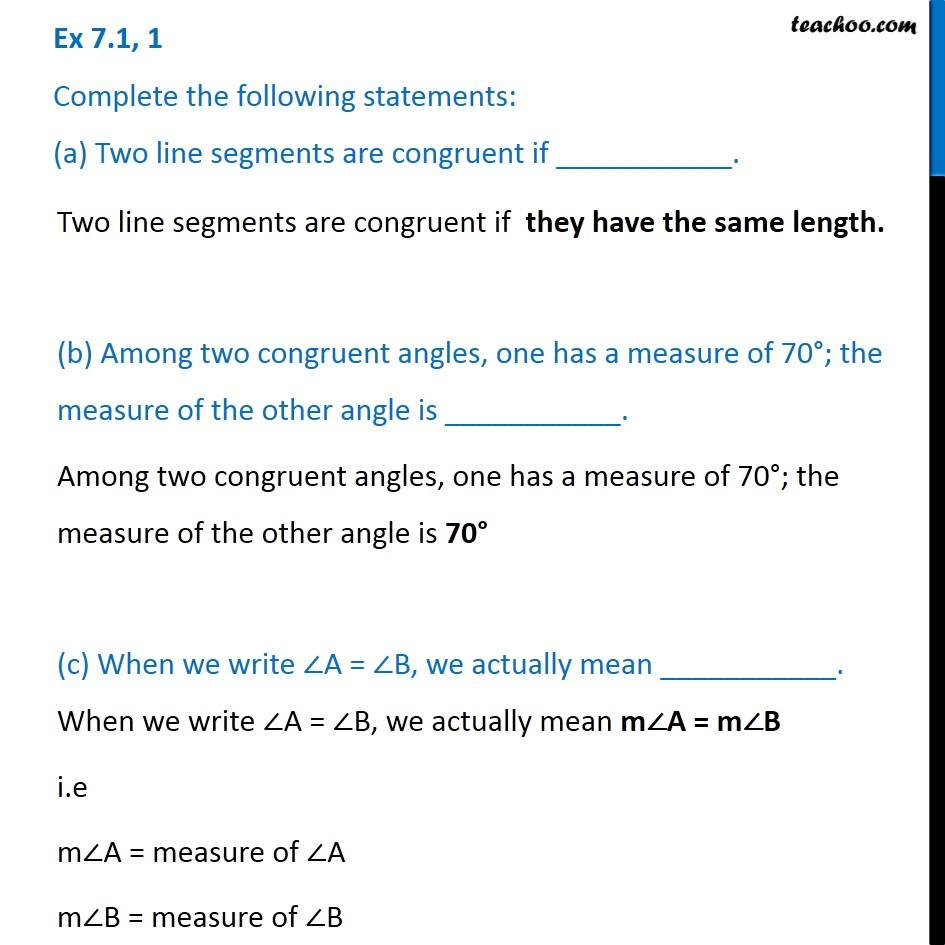1. Chapter 7 Class 7 Congruence of Triangles
2. Concept wise
3. Congruency

Transcript

Ex 7.1, 1 Complete the following statements: (a) Two line segments are congruent if ___________. Two line segments are congruent if they have the same length. (b) Among two congruent angles, one has a measure of 70°; the measure of the other angle is ___________. Among two congruent angles, one has a measure of 70°; the measure of the other angle is 70° (c) When we write ∠A = ∠B, we actually mean ___________. When we write ∠A = ∠B, we actually mean m∠A = m∠B i.e m∠A = measure of ∠A m∠B = measure of ∠B